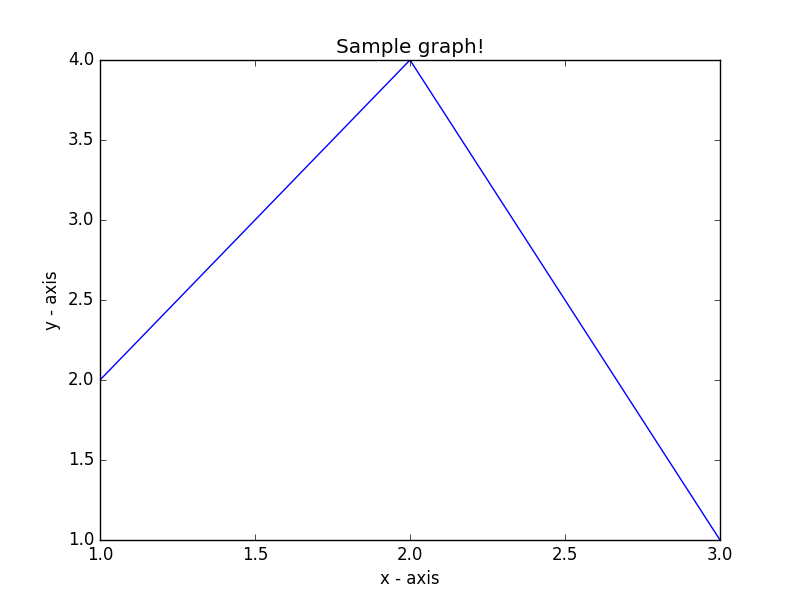﻿ Matplotlib Basic: Draw a line using given axis values with suitable label in the x axis , y axis and a title - w3resource# Matplotlib Basic: Draw a line using given axis values with suitable label in the x axis , y axis and a title

## Matplotlib Basic: Exercise-2 with Solution

Write a Python program to draw a line using given axis values with suitable label in the x axis , y axis and a title.

Sample Solution:

Python Code:

``````import matplotlib.pyplot as plt
# x axis values
x = [1,2,3]
# y axis values
y = [2,4,1]
# Plot lines and/or markers to the Axes.
plt.plot(x, y)
# Set the x axis label of the current axis.
plt.xlabel('x - axis')
# Set the y axis label of the current axis.
plt.ylabel('y - axis')
# Set a title
plt.title('Sample graph!')
# Display a figure.
plt.show()
```
```

Sample Output:Python Code Editor: